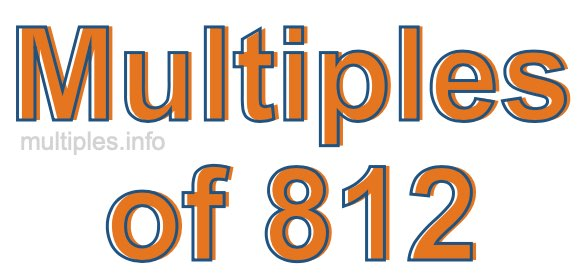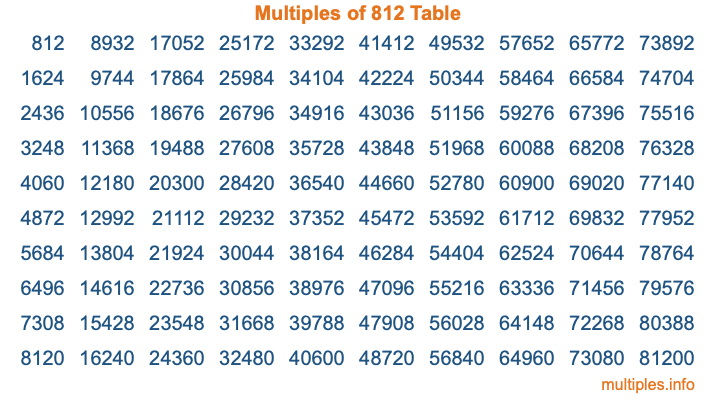Multiples of 812Welcome to the Multiples of 812 page. Here we will first teach you everything you will ever need to know about the multiples of 812, and then give you a study guide summary of everything we taught you to make sure you remember it all. Use this page to look up facts and learn information about the multiples of 812. This page will make you a multiples of eight hundred twelve expert!

Definition of Multiples of 812
Multiples of 812 are all the numbers that when divided by 812 equal an integer. Each of the multiples of 812 are called a multiple. A multiple of 812 is created by multiplying 812 by an integer.

Therefore, to create a list of multiples of 812, you start with 1 multiplied by 812, then 2 multiplied by 812, then 3 multiplied by 812, and so on for as long as you want. Thus, the list of the first five multiples of 812 is 812, 1624, 2436, 3248, and 4060. To see a larger list of multiples of 812, see the printable image of Multiples of 812 further down on this page. We also have a category where you can choose any nth multiple of 812.

Multiples of 812 Checker
The Multiples of 812 Checker below checks to see if any number of your choice is a multiple of 812. In other words, it checks to see if there is any number (integer) that when multiplied by 812 will equal your number. To do that, we divide your number by 812. If the the quotient is an integer, then your number is a multiple of 812.

Is  a multiple of 812?

Least Common Multiple of 812 and ...
A Least Common Multiple (LCM) is the lowest multiple that two or more numbers have in common. This is also called the smallest common multiple or lowest common multiple and is useful to know when you are adding our subtracting fractions. Enter one or more numbers below (812 is already entered) to find the LCM.

Check out our LCM Calculator if you need more details about the Least Common Multiple or if you need the LCM for different numbers for adding and subtraction fractions.

nth Multiple of 812
As we stated above, 812 is the first multiple of 812, 1624 is the second multiple of 812, 2436 is the third multiple of 812, and so on. Enter a number below to find the nth multiple of 812.

th multiple of 812

Multiples of 812 vs Factors of 812
812 is a multiple of 812 and a factor of 812, but that is where the similarities end. All postive multiples of 812 are 812 or greater than 812. All positive factors of 812 are 812 or less than 812.

Below is the beginning list of multiples of 812 and the factors of 812 so you can compare:

Multiples of 812: 812, 1624, 2436, 3248, 4060, etc.

Factors of 812: 1, 2, 4, 7, 14, 28, 29, 58, 116, 203, 406, 812

As you can see, the multiples of 812 are all the numbers that you can divide by 812 to get a whole number. The factors of 812, on the other hand, are all the whole numbers that you can multiply by another whole number to get 812.

It's also interesting to note that if a number (x) is a factor of 812, then 812 will also be a multiple of that number (x).

Multiples of 812 vs Divisors of 812
The divisors of 812 are all the integers that 812 can be divided by evenly. Below is a list of the divisors of 812.

Divisors of 812: 1, 2, 4, 7, 14, 28, 29, 58, 116, 203, 406, 812

The interesting thing to note here is that if you take any multiple of 812 and divide it by a divisor of 812, you will see that the quotient is an integer.

Multiples of 812 Table
Below is an image of the first 100 multiples of 812 in a table. The table is in chronological order, column by column. The first column has the first ten multiples of 812, the second column has the next ten multiples of 812, and so on.The Multiples of 812 Table is also referred to as the 812 Times Table or Times Table of 812. You are welcome to print out our table for your studies.

Negative Multiples of 812
Although not often discussed or needed in math, it is worth mentioning that you can make a list of negative multiples of 812 by multiplying 812 by -1, then by -2, then by -3, and so on, to get the following list of negative multiples of 812:

-812, -1624, -2436, -3248, -4060, etc.

Multiples of 812 Summary
Below is a summary of important Multiples of 812 facts that we have discussed on this page. To retain the knowledge on this page, we recommend that you read through the summary and explain to yourself or a study partner why they hold true.

There are an infinite number of multiples of 812.

A multiple of 812 divided by 812 will equal a whole number.

812 divided by a factor of 812 equals a divisor of 812.

The nth multiple of 812 is n times 812.

The largest factor of 812 is equal to the first positive multiple of 812.

812 is a multiple of every factor of 812.

812 is a multiple of 812.

A multiple of 812 divided by a divisor of 812 equals an integer.

812 divided by a divisor of 812 equals a factor of 812.

Any integer times 812 will equal a multiple of 812.

Multiples of a Number
Here you can get the multiples of another number, all with the same attention to detail as we did for multiples of 812 on this page.

Multiples of
Multiples of 813
Did you find our page about multiples of eight hundred twelve educational? Do you want more knowledge? Check out the multiples of the next number on our list!